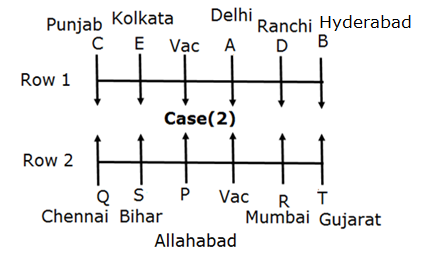# IBPS PO Mains Reasoning Questions 2019 – (Day-4)

Dear Aspirants, Our IBPS Guide team is providing new pattern Reasoning Questions for IBPS PO Mains 2019 so the aspirants can practice it on a daily basis. These questions are framed by our skilled experts after understanding your needs thoroughly. Aspirants can practice these new series questions daily to familiarize with the exact exam pattern and make your preparation effective.

Check here for IBPS PO Mains Mock Test 2019

[WpProQuiz 7290]

Puzzle

Directions (1-5): Study the following information carefully and answer the below questions.

There is a 5×5 matrix which can produce string signals to operate the traffic signals of Green, Red and Yellow light. The rows of the matrix are =, @, #, %, and \$ from bottom to top and the columns are A, B, C, D and E from left to right end.

Top row contains numbers which are consecutive multiple of 2 from 12. Third row from the bottom contains numbers which are consecutive multiple of 5 from 15. Second row from the bottom contains numbers which are consecutive multiple of 9 from 27.  Second row from the top contains numbers which are consecutive number of following equation n(n+1)/2 starting from n=3. Bottom row contains numbers which are consecutive perfect square number starting from 4.

The matrix numeric produce the signal to operate the lights such as., If the numeric value of string is between 20 to 45 then Red lights on. If the value is between 50 and 95 then Orange lights is on. If the value is between 100 and 165 then Yellow lights is on. If the value is between 170 and 300 then Green is operate. Except these values the lights does not operate.

Conditions for Numeric signal values are,

If 1st and last number is a perfect square from even numbers, then the 10th digits of all numbers are multiplied to produce numeric values.

If perfect square is preceded to odd number, then sum the highest and lowest number.

If perfect square is preceded to even number then subtract then 2nd highest and 2nd lowest number.

If perfect cube is preceded to perfect square number then sum the highest and lowest number.

There is no conditions followed then sum the all one’s digits. If two conditions are follow in the signal order wise 1st follow condition only applicable.

1) If signals 1 -> ‘\$A%C#[email protected]=E’, 2 -> ‘\$D%B#[email protected]=E’, 3 -> ‘\$D%C#[email protected]=B’ to form signal ‘1+2+3’ then which of the following light is operated?

a) Yellow

b) Red

c) Green

d) Orange

e) None of these

2) If the signal ‘\$D%E#[email protected]=C’, then which of the light is operated?

a) Red

b) Orange

c) Yellow

d) Green

e) None of these

3) If the signal ‘\$A%C#[email protected]=A’, then which of the light is operated?

a) Red

b) Yellow

c) Orange

d) Green

e) None of these

4) In the given following which signal value is correct to operate the Yellow light?

a) \$A%B#[email protected]=D

b) \$C%E#[email protected]=E

c) @D\$E#A%B=C

d) All signals are operate

e) None of these

5) Signals A is ‘=E%E#[email protected]\$C’, B is ‘=E%[email protected]\$E\$C’, if ‘A+B’ which of the following light will be operated?

a) Yellow

b) Orange

c) Red

d) Green

e) Cannot be determined

Seating arrangement

Directions (6-10): Study the following information carefully and answer the below questions.

Ten persons are sitting in a parallel row with equidistance between them. Each row has six seats including vacant seat. In row 1 – A, B, C, D and E are sitting by facing south and in row 2 – P, Q, R, S and T are sitting facing north. In the given seating arrangement each person seated in the row faces another row seated persons or vacant seat. All of them are from different cities, viz. Chennai, Mumbai, Ranchi, Bihar, Kolkata, Delhi, Allahabad, Gujarat and Hyderabad but not in the same order.

S is an immediate neighbour of P and Q. The one who is from Mumbai faces the person who is from Ranchi. The person from Bihar sits third to the left of the one from Mumbai. The vacant seat of the first row is on the immediate left of one who faces the person from Bihar. The one from Kolkata sits third to the right of the one who faces the person from Mumbai. Only two persons sit between Q and the vacant seat. B sits on the immediate left of the one from Ranchi. The one who is from Punjab is not a neighbour of the one from Ranchi. Q faces C, who sits third to the right of A and D is one of the immediate neighbour of A. The person from Delhi sits on the immediate left of the vacant seat of the 1st row. The vacant position of 1st row faces the one from Allahabad. T sits on a extreme end of the row and he is from Gujarat. The one who faces T is an immediate neighbour of the person from Ranchi. The person from Allahabad doesn’t face south. The person neither from Hyderabad nor from Punjab faces north.

6) Which of the following statement is not true?

a) The person from Bihar sits on the immediate left of P and immediate right of Q

b) The person from Hyderabad and Chennai sits diagonally opposite each other.

c) The person from Allahabad sit 2nd to the right of the person who faces T.

d) The person from Chennai sits on the immediate left of the person who faces E.

e) None of these

7) How many persons sits between vacant seat and Hyderabad?

a) Three

b) Five

c) Four

d) Two

e) None of these

8) Who sits 3rd to the left of the one who faces Chennai?

a) Person from Ranchi

b) D

c) A

e) None of these

9) Four of the following five are alike in a certain way. Which one does not belong to the group?

a) Punjab-T

c) Chennai-B

d) Ranchi-R

e) Gujarat- C

10) Who sits immediate left of vacant seat in row 2?

a) P

b) R

c) T

d) Q

e) None of these

Directions (1-5):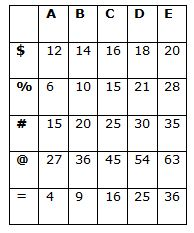There is a 5×5 matrix which can produce string signals to operate the traffic signals of Green, Red and Yellow light. The rows of the matrix are =, @, #, %, and \$ from bottom to top and the columns are A, B, C, D and E from left to right end.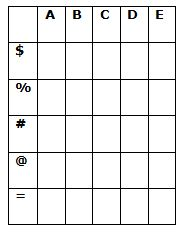Top row contains numbers which are consecutive multiple of 2 from 12. In the statement consecutive multiple of 2 from 12 onwards is 12, 14, 16, 18 and 20.

Second row from the top contains numbers which are consecutive number of following equation (n(n+1)/2) starting from n=3. We set n as 3 and 4 and so on for the values.

Third row from the bottom contains numbers which are consecutive multiple of 5 from 15. We get values 15, 20, 25, 30 and 35 same method as 1st row from top.

Second row from the bottom contains numbers which are consecutive multiple of 9 from 27 so the values are 27, 36, 45, 54 and 63.

Bottom row contains numbers which are consecutive perfect square number starting from 4. Perfect square values from 4 onwards viz. 4, 9, 16, 25 and 36.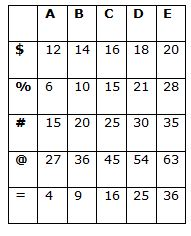1=\$A%C#[email protected]=E, 2= \$D%B#[email protected]=E, 3=\$D%C#[email protected]=B

1= 12 15 25 54 36 from this condition 3 is followed. Perfect square number is preceded by even number. So, subtract the second highest and 2rd lowest.

36-15=21

2= 18 10 25 45 36 from this condition 2 is followed. Perfect square is preceded by odd number, then sum the highest and lowest number.

45+10=55

3= 18 15 30 36 9 from this condition 2 is followed. Perfect square is preceded by odd number, then sum the highest and lowest number.

36+9=45

1+2+3 -> 21+55+45 -> 121. So, Yellow light is on.

‘\$D%E#[email protected]=C’ is 18 28 35 27 16 from this the perfect cube is preceded by perfect square number. So, sum the highest and lowest number.

35+16= 51. Since, the value is between 50 and 95 So, the Orange lights is on.

Signal ‘\$A%C#[email protected]=A’ is 12 15 20 54 4 form this condition 3 is followed. Perfect square number is preceded by even number. So, subtract the second highest and 2rd lowest.

20 -12 = 8, So, no light is operated.

‘\$C%E#[email protected]=E’ is 16 28 35 63 36. 1st and last number is a perfect square from even numbers. So, the 10th digits of all numbers are multiplied to produce numeric values.

1x2x3x6x3 = 108 Yellow light has been operated by this signal.

If two conditions are follow in the signal order, then only 1st condition which follows will be applicable.

Signals A is =E%E#[email protected]\$C is 36 28 30 54 16. 1st and last number is a perfect square from even numbers. So, the 10th digits of all numbers are multiplied to produce numeric values.

3x2x3x5x1= 90

Signals B is =E%[email protected]\$E\$C is 36 28 63 20 16. 1st and last number is a perfect square from even numbers. So, the 10th digits of all numbers are multiplied to produce numeric values.

3x2x6x2x1 = 72

90+72 = 162. So, Yellow light is operated.

Directions (6-10):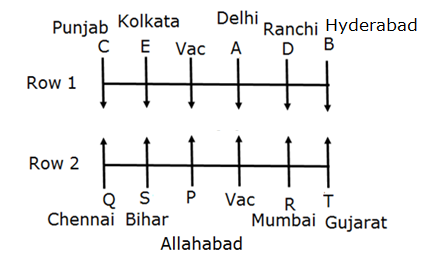The person from Bihar sits third to the left of the one from Mumbai. The vacant seat of the first row is on the immediate left of one who faces the person from Bihar. The one from Kolkata sits third to the right of the one who faces the person from Mumbai.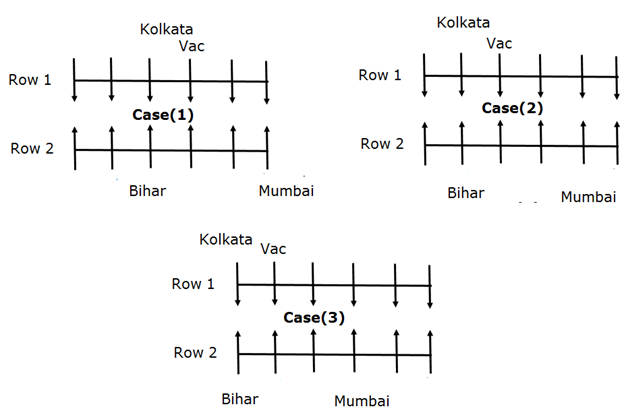The person from Delhi sits on the immediate left of the vacant seat of the 1st row. The vacant position of 1st row faces the one from Allahabad. The person from Allahabad doesn’t face south.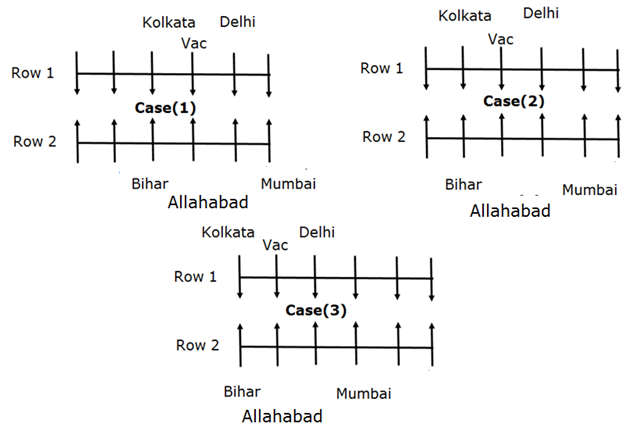The one who is from Mumbai faces the person who is from Ranchi. T sits on a extreme end of the row and he is from Gujarat. The one who faces T is an immediate neighbour of the person from Ranchi.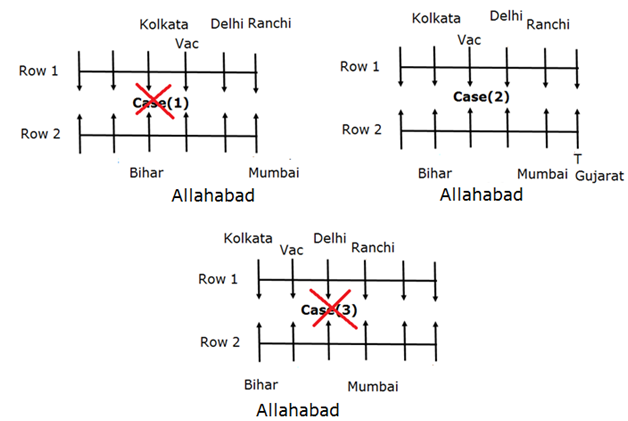Case (1) and Case (3) gets eliminated because we have no place to fix the position of T.

B sits on the immediate left of the one from Ranchi. Q faces C, who sits third to the right of A and D is one of the immediate neighbour of A.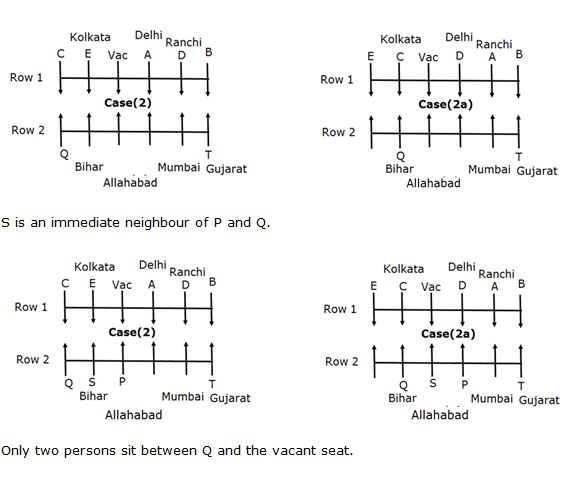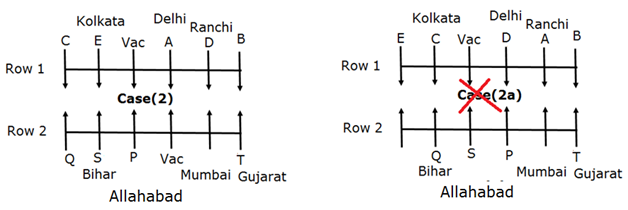Case (2a) gets eliminated because we have no place for Vacant.

The person neither from Hyderabad nor from Punjab faces north. The one who is from Punjab is not a neighbour of the one from Ranchi.